# Decoding Strategies that You Need to Know for Response Generation

## Distinguish between Beam Search, Random Sampling, Top-K, and Nucleus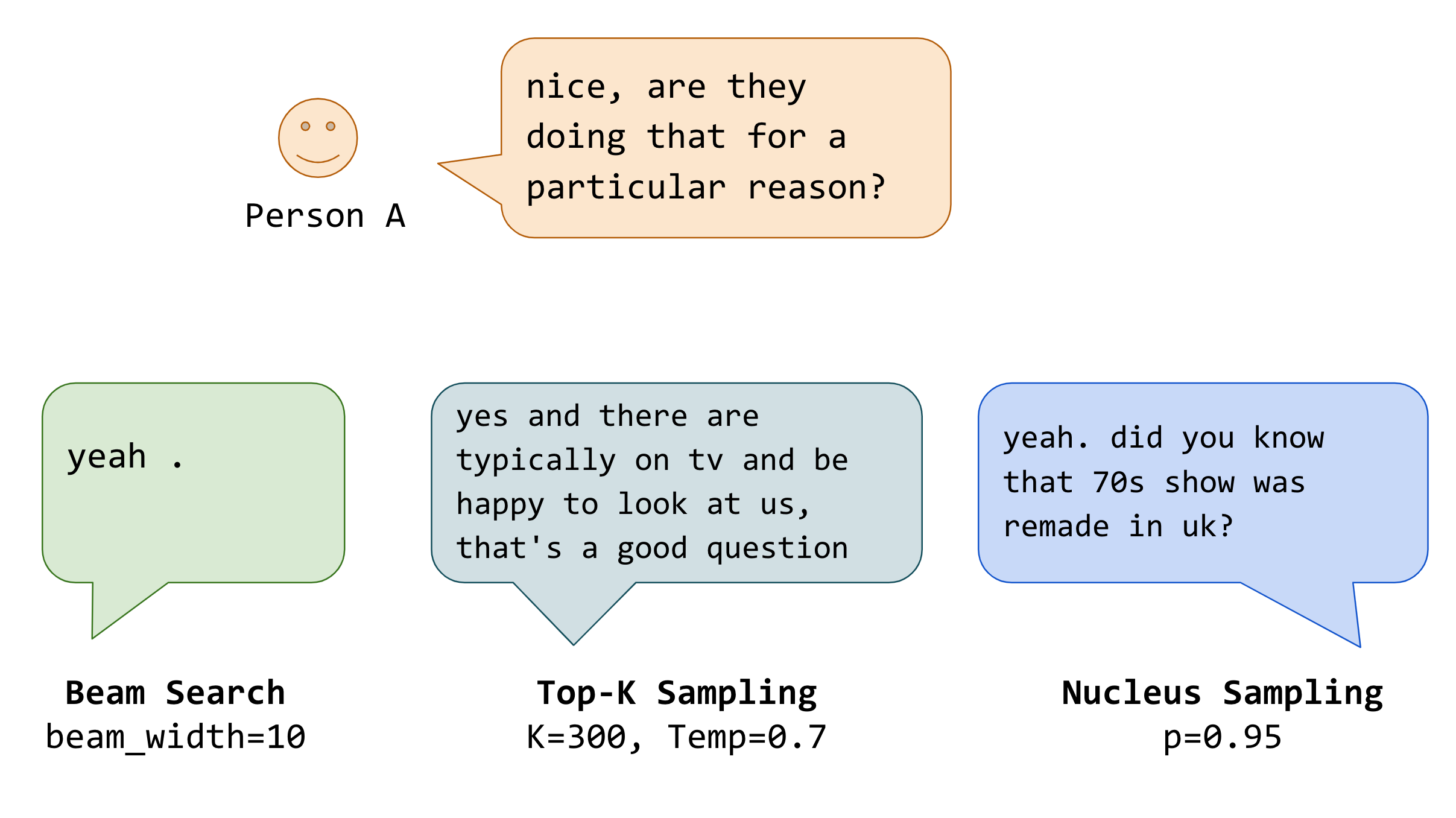Different Sampling Techniques. Note, we did not use strong generative model for this example.

# Introduction

Deep learning has been deployed in many tasks in NLP, such as translation, image captioning, and dialogue systems. In machine translation, it is used to read source language (input) and generate the desired language (output). Similarly in a dialogue system, it is used to generate a response given a context. This is also known as Natural Language Generation (NLG).

The model splits into 2 parts: encoder and decoder. Encoder reads the input text and returns a vector representing that input. Then, the decoder takes that vector and generates a corresponding text.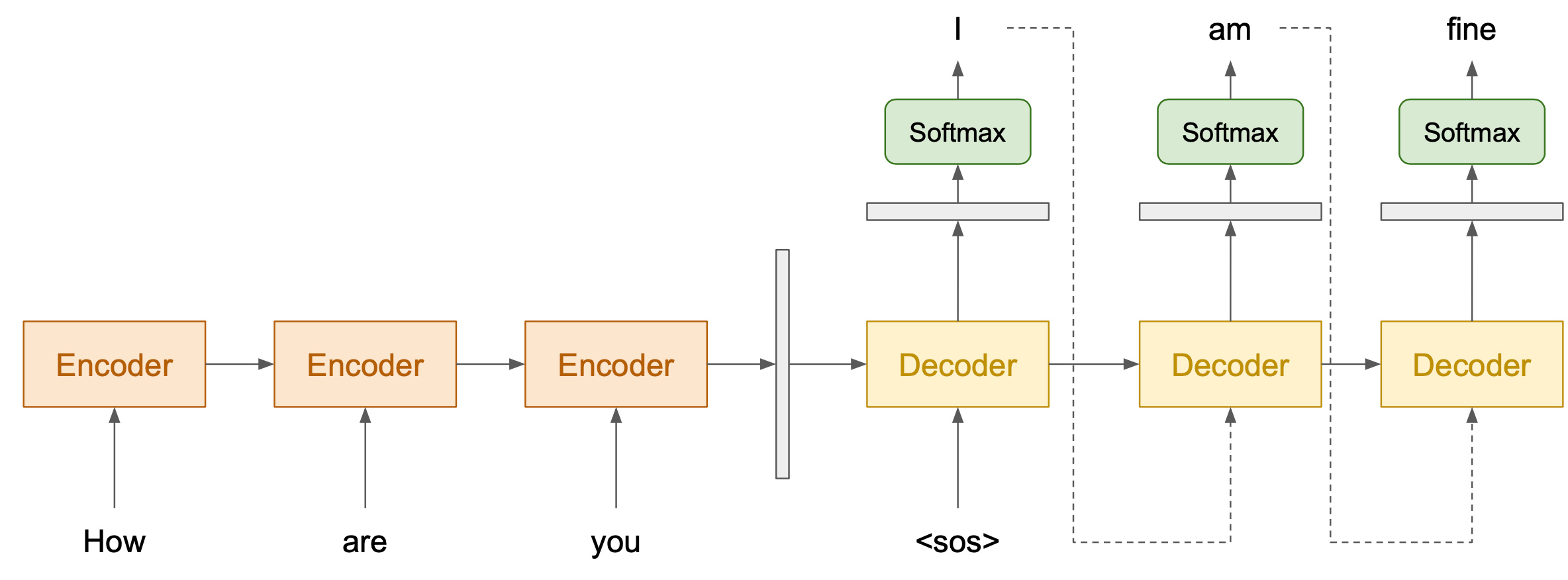Figure 1: Encoder-Decoder Architecture

To generate a text, commonly it is done by generating one token at a time. Without proper techniques, the generated response may be very generic and boring. In this article, we will explore the following strategies:

• Greedy
• Beam Search
• Random Sampling
• Temperature
• Top-K Sampling
• Nucleus Sampling

# Decoding Strategies

At each timestep during decoding, we take the vector (that holds the information from one step to another) and apply it with softmax function to convert it into an array of probability for each word.Equation 1: Softmax Function. x is a token at timestep i. u is the vector that contains the value of every token in the vocabulary.

This approach is the simplest. At each time-step, it just chooses whichever token that is the most probable.

Context:            Try this cake. I baked it myself.Optimal Response  : This cake tastes great.Generated Response: This is okay.

However, this approach may generate a suboptimal response, as shown in the example above. The generated response may not be the best possible response. This is due to the training data that commonly have examples like “That is […]”. Therefore, if we generate the most probable token at a time, it might output “is” instead of “cake”.

Exhaustive search can solve the previous problem since it will search for the whole space. However, it would be computationally expensive. Suppose there are 10,000 vocabularies, to generate a sentence with the length of 10 tokens, it would be (10,000)¹⁰.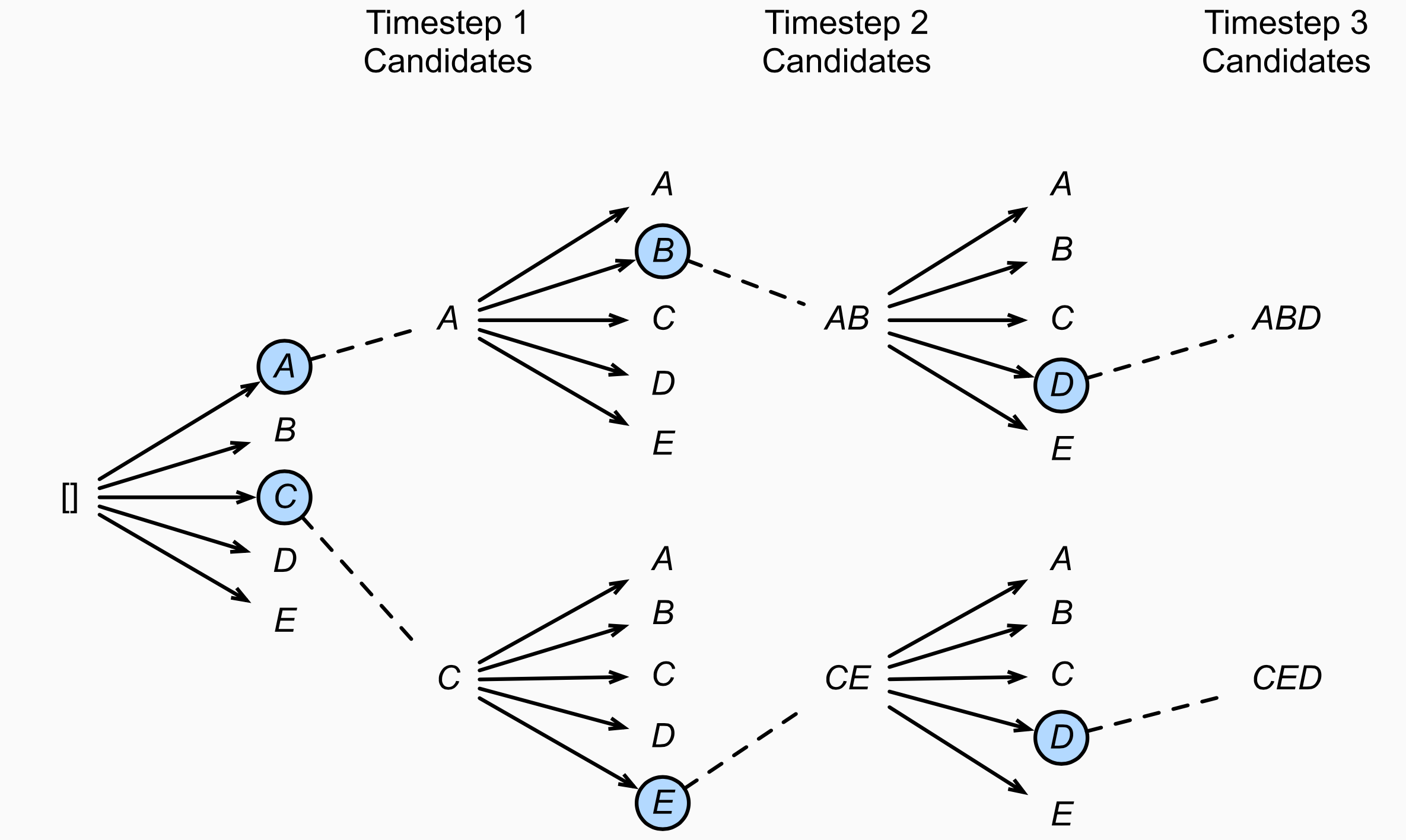Figure 2: Beam Search with BeamWidth=2 (Source)（束宽为2，输出序列最大长度为3。候选输出序列有A、C、AB、CE、ABD和CED）

Beam search can cope with this problem. At each timestep, it generates all possible tokens in the vocabulary list; then, it will choose top B candidates that have the most probability. Those B candidates will move to the next time step, and the process repeats. In the end, there will only be B candidates. The search space is only (10,000)*B.

Context:    Try this cake. I baked it myself.Response A: That cake tastes great.Response B: Thank you.

But sometimes, it chooses an even more optimal (Response B). In this case, it makes perfect sense. But imagine that the model likes to play safe and keeps on generating “I don’t know” or “Thank you” to most of the context, that is a pretty bad bot.

https://tangshusen.me/Dive-into-DL-PyTorch/#/chapter10_natural-language-processing/10.10_beam-search

Alternatively, we can look into stochastic approaches to avoid the response being generic. We can utilize the probability of each token from the softmax function to generate the next token.

Suppose we are generating the first token of a context “I love watching movies”, Figure below shows the probability of what the first word should be.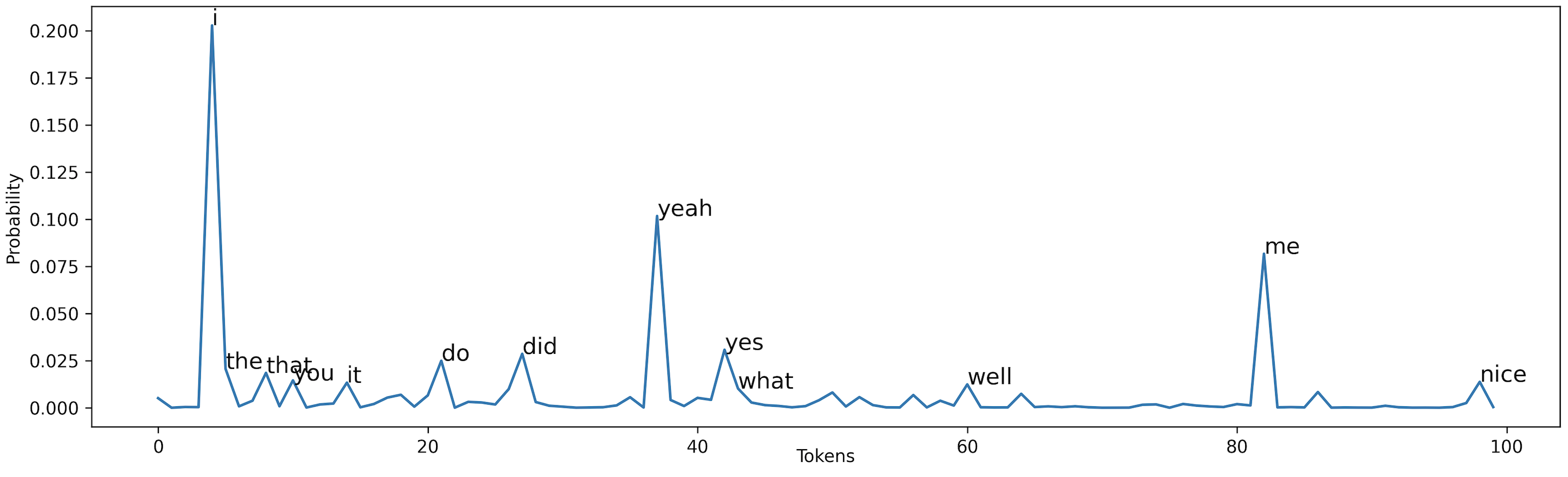Figure 3: Probability of each word. X-axis is the token index. i.e, index 37 corresponds to the word “yeah”

If we use a greedy approach, a token “i” will be chosen. With random sampling, however, token i has a probability of around 0.2 to occur. At the same time, any token that has a probability of 0.0001 can also occur. It’s just very unlikely.

Random sampling, by itself, could potentially generate a very random word by chance. Temperature is used to increase the probability of probable tokens while reducing the one that is not. Usually, the range is 0 < temp ≤ 1. Note that when temp=1, there is no effect.Equation 2: Random sampling with temperature. Temperature t is used to scale the value of each token before going into a softmax function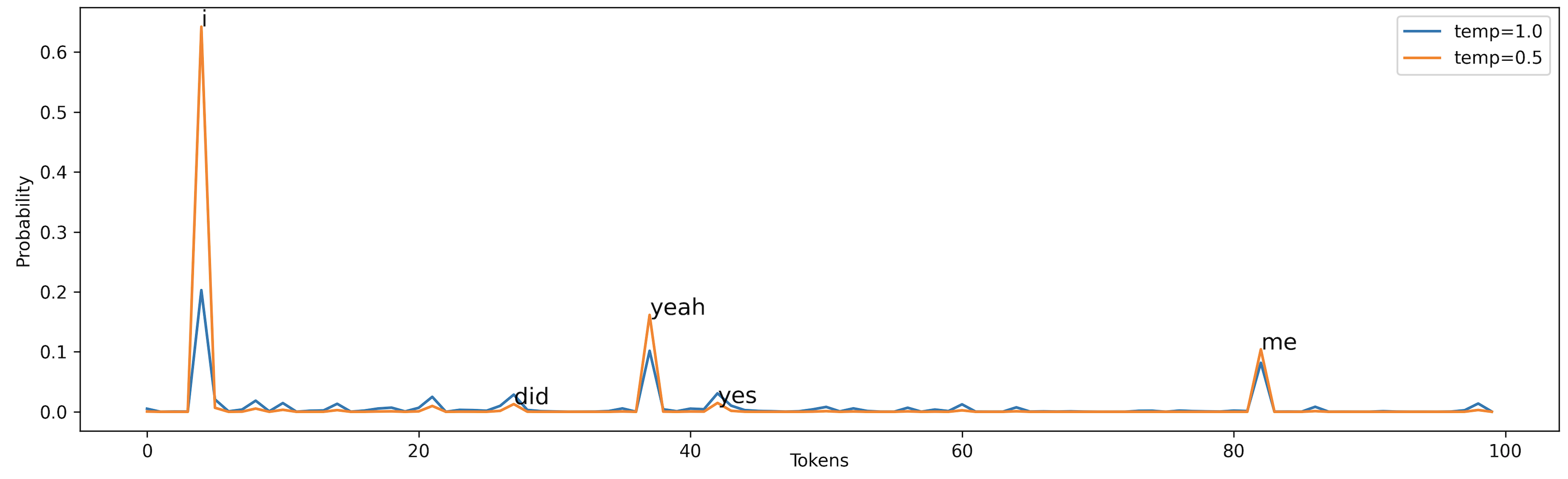Figure 4: Random sampling vs. random sampling with temperature

In Figure 4, with temp=0.5, the most probable words like iyeahme, have more chance of being generated. At the same time, this also lowers the probability of the less probable ones, although this does not stop them from occurring.

top-k的思想是让预测概率很低的词完全没有机会输出，采样前按概率分布截断，只考虑从预测概率高的前top-k个词中选择输出词。top-k之后的词概率设为0

Top-K sampling is used to ensure that the less probable words should not have any chance at all. Only top K probable tokens should be considered for a generation.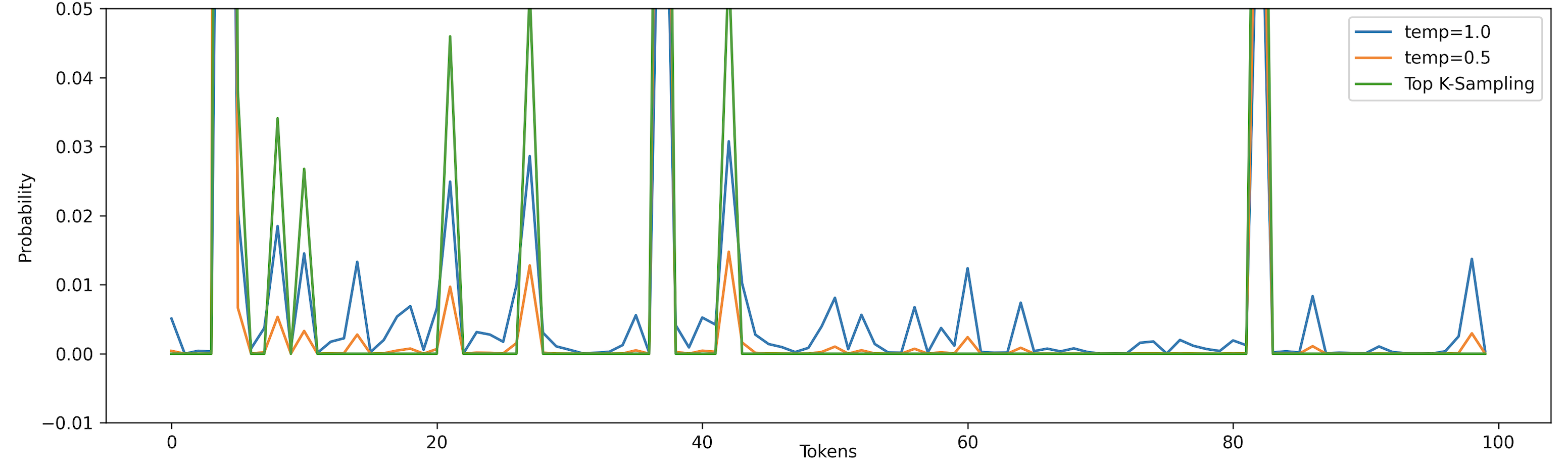Figure 5: Distribution of the 3 random sampling, random with temp, and top-k

The token index between 50 to 80 has some small probabilities if we use random sampling with temperature=0.5 or 1.0. With top-k sampling (K=10), those tokens have no chance of being generated. Note that we can also combine Top-K sampling with temperature, but you kinda get the idea already, so we choose not to discuss it here.

This sampling technique has been adopted in many recent generation tasks. Its performance is quite good. One limitation with this approach is the number of top K words need to be defined in the beginning. Suppose we choose K=300; however, at a decoding timestep, the model is sure that there should be 10 highly probable words. If we use Top-K, that means we will also consider the other 290 less probable words.

核采样想解决top-k k不好找的问题，他的目的是想找到一个最小的候选集合，该候选集的概率密度之和大于某个阈值p。同样的，选出来的候选集里每个词的概率还要重新归一化，让其和为1。集合外的其他词概率设为0.

Nucleus sampling is similar to Top-K sampling. Instead of focusing on Top-K words, nucleus sampling focuses on the smallest possible sets of Top-V words such that the sum of their probability is ≥ p. Then, the tokens that are not in V^(p) are set to 0; the rest are re-scaled to ensure that they sum to 1.Equation 3: Nucleus sampling. V^(p) is the smallest possible of tokens. P(x|…) is the probability of generating token x given the previous generated tokens x from 1 to i-1

The intuition is that when the model is very certain on some tokens, the set of potential candidate tokens is small otherwise, there will be more potential candidate tokens.

Certain → those few tokens have high probability = sum of few tokens is enough to exceed p.
Uncertain → Many tokens have small probability = sum of many tokens is needed to exceed p.Figure 6: Distribution of Top-K and Nucleus Sampling

Comparing nucleus sampling (p=0.5) with top-K sampling (K = 10), we can see the nucleus does not consider token “you” to be a candidate. This shows that it can adapt to different cases and select different numbers of tokens, unlike Top-K sampling.

# Summary

• Greedy: Select the best probable token at a time
• Beam Search: Select the best probable response
• Random Sampling: Random based on probability
• Temperature: Shrink or enlarge probabilities
• Top-K Sampling: Select top probable K tokens
• Nucleus Sampling: Dynamically choose the number of K (sort of)

Commonly, top choices by researchers are beam search, top-K sampling (with temperature), and nucleus sampling.

# Conclusion

We have gone through a list of different ways to decode a response. These techniques can be applied to different generation tasks, i.e., image captioning, translation, and story generation. Using a good model with bad decoding strategies or a bad model with good decoding strategies is not enough. A good balance between the two can make the generation a lot more interesting.

# References

Holtzman, A., Buys, J., Du, L., Forbes, M., & Choi, Y. (2020). The Curious Case of Neural Text Degeneration. In International Conference on Learning Representations.

posted @ 2020-12-26 19:32  fnangle  阅读(262)  评论(0编辑  收藏  举报